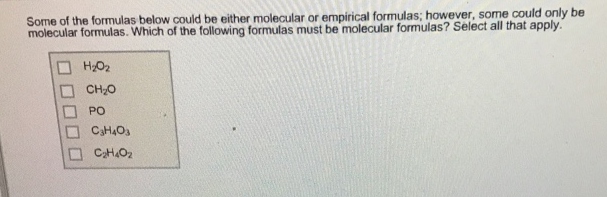# Problem: Some of the formulas below could be either molecular or empirical formulas; however H2O2CH2OPOC3H4O3C2H4O2

###### FREE Expert Solution

Empirical formula is the simplest positive integer ratio of atoms present in a compound.

H2O(Incorrect; this can be simplified to HO)\

82% (499 ratings)###### Problem Details

Some of the formulas below could be either molecular or empirical formulas; however

H2O2

CH2O

PO

C3H4O3

C2H4O2Frequently Asked Questions

What scientific concept do you need to know in order to solve this problem?

Our tutors have indicated that to solve this problem you will need to apply the Empirical Formula concept. You can view video lessons to learn Empirical Formula. Or if you need more Empirical Formula practice, you can also practice Empirical Formula practice problems.